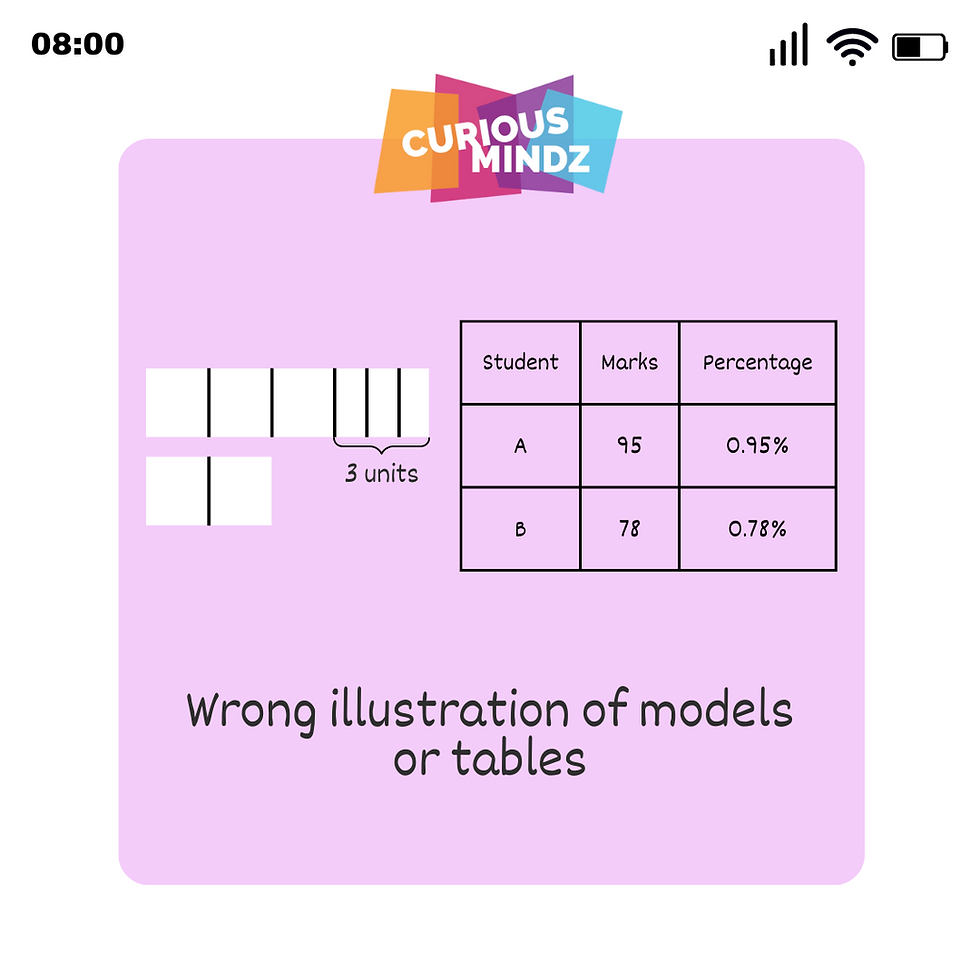top of page
Search

# Mathematical mistakes to avoid

Updated: Sep 29, 2022Doing Math questions may be challenging and overwhelming but sometimes some small mistakes made can be avoided to allow you to score better in your Math tests and exams.

Here are some ways to avoid some mistakes that are commonly made by students.##### Underline key information

For example,

At Mr. Tan’s fruit stall, 250 oranges were sold in the morning and 3/4 of the remaining oranges were sold in the afternoon. As a result, 1/6 of the total number of oranges at first were left. If the oranges were sold at \$2.80 in packs of 5 oranges, how much did Mr. Tan receive from the sales of the oranges in the afternoon

PSLE Math is known to be tricky and sometimes, the questions will trick you into applying the wrong concepts. This tip is helpful for you to be able to see the main points more clearly. Being able to see and understand what the question wants, can aid you in getting the right strategies or methods to solve the question quicker with more accuracy.##### Be mindful of your units

For example,

The distance between points A and B is 10.8 km, and Hugo runs at a speed of 2 m/s. How long does it take to run from point A to B? Give your answers in terms of hours.

Time = Distance/Speed

Distance = 10.8 km = 10800 m

Speed = 2 m/s

Substituting Distance and Speed into the equation,

Time = 10800/2

Time = 5400 s

Time (in hours) = 5400 ÷ 3600 = 1.5 h

This tip is important, especially in topics such as ‘Distance, Time and Speed’, ‘Length, Mass and Volume’ and ‘Areas and Perimeters’, as unit conversions are usually required. It will be good to circle the units used in the questions to serve as a reminder to you to convert your units while attempting to solve the question.

1 cm = 0.01 m = 0.00001 km

1 cm2 = 0.001 m^2

1 cm3 = 0.000001 m^3 = 1 mL = 0.001L

1 h = 60 min = 3600 sFor example, ‘There were 150 red apples and 90 green apples in a basket. What percentage of the total number of apples were green?Workings should be presented in a neat and logical flow so that the marker will be able to understand your train of thought and know where to give you your method marks. This is especially important when dealing with fractions and percentages.

You can improve this by presenting your solutions properly while doing your daily practice.##### Memorise essential formulas

Have you ever known what formula you need to use during your exam, but are unable to recall what it is?

This happens to many students. To help you memorise your formulas, constantly test your memory and apply these formulas to questions without any reference to your notes. With enough practice before exams, you will be able to remember the formulas by heart and apply them without any difficulties.

Additionally, during your revision, you can create a list of formulas that are required and only refer to them when you cannot remember the formulas or you can read through the formulas once every day to recap.##### Apply order of operations correctly

For example,

Find the value of 20 - 8 ÷ 4 x (2+6) + 1.

20 - 8 ÷ 4 x (2+6) + 1

= 20 - 8 ÷ 4 x (8) + 1 (do operations within the brackets)

= 20 - 2 x 8 + 1 (do multiplication and/or division from left to right)

= 20 - 16 + 1 (do addition and/or subtractions from left to right)

= 4 + 1

= 5

This is an important thing to take note of as you may be tested on your order of operations in some questions. The order of operation is

1. Do all the operations within the brackets.

2. Do multiplications and/or division from left to right.

3. Do addition and/or subtraction from left to right.

It will be good to practice it frequently to help you solve your questions faster and with more ease.##### Illustrate models or tables

You are taught to use tables or models to solve problem sums. It is good to use them to answer questions as they can help you better visualise the questions and information given, and help you solve certain questions with higher accuracy.

You can practice this by doing more higher-order thinking questions that test you on your question interpretations and how you use the information given to draw models or tables to solve the questions.7. Reduce careless mistakes

Careless mistakes are avoidable. This is one of the most common PSLE Math mistakes that most students make. Miscalculations, writing or transferring the incorrect number are things that usually happen. However, they are easy to avoid.

You can avoid having miscalculations by using a calculator to check through your workings once more before proceeding to the next question or learning not to skip important steps in the calculation process. Also, you can avoid transferring the incorrect number by reading the question twice and being confident about your plan to solve the question before starting it. Sometimes you may read numbers like 51 like 15 or 61 as 91 as you are rushing to complete your paper, hence causing you to lose more marks.

Hope these tips will help and remind you to make less of such mistakes. Happy learning!